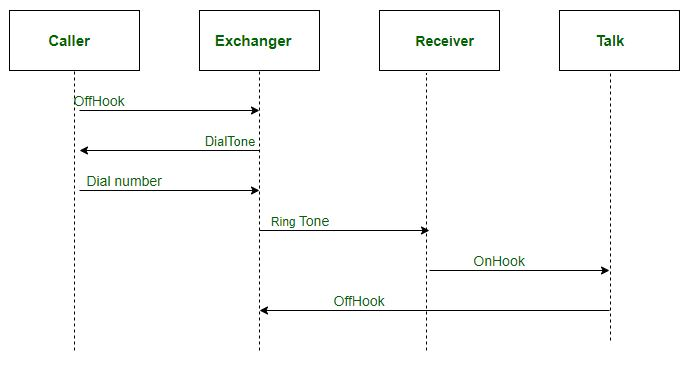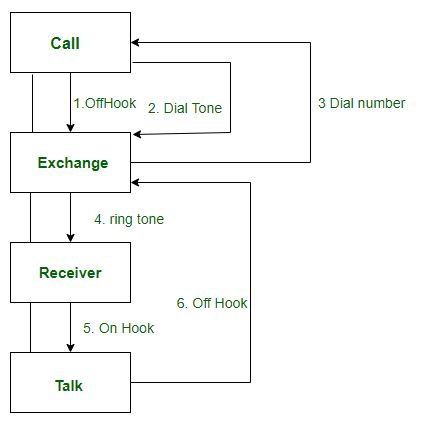Related Articles

# Difference between Sequence diagram and Collaboration diagram

• Difficulty Level : Hard
• Last Updated : 22 Oct, 2020

A Sequence diagram is an interaction diagram that details about the operation that is carried out. The sequence diagram captures the interaction between the objects in the context of collaboration. Sequence diagrams are time focused and they show the order of the interaction visually by using the vertical axis of the diagram to represent time.

Example:Collaboration Diagram represents the interaction of the objects to perform the behavior of a particular use case or a part of use case. The designers use the Sequence diagram and Collaboration Diagrams to define and clarify the roles of the objects that perform a particular flow of events of a use case.

Example:Similarities Between Sequence and Collaboration Diagram

1. In Unified Modelling Language both the sequence diagram and collaboration diagram are used as interaction diagrams.
2. Both the diagrams details about the behavioral aspects of the system.

Differences Between Sequence and Collaboration diagram:

My Personal Notes arrow_drop_up